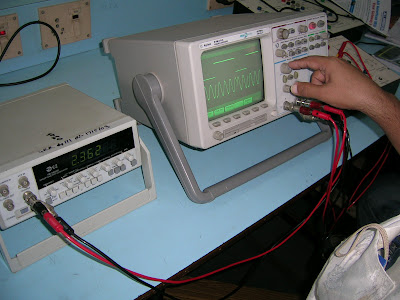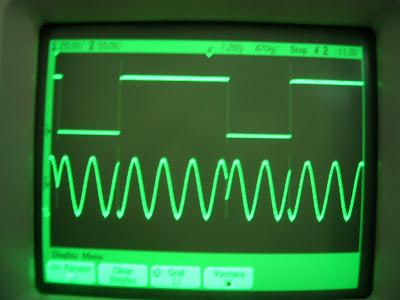### Binary Phase Shift Keying

Here are some photos and waveforms from a PSK generation lab practicals. All undergraduate students pursuing engineering in electronics and its allied fields get a chance to do it at least once during their four years.
The process of PSK generation in a lab is as follows:

1. Generate a sinusoidal carrier wave from a signal generator. Call it Signal A
2. Use an operational amplifier to shift the phase of Signal A by a fixed amount : usually 90 degrees in case of Binay Phase Shift Keying. Call this Signal B.
3. Apply Signal A and Signal B to the two inputs of a 2:1 analog multiplexer.
4. Apply the data signal to the select line of the analog switch. The output of the analog switch will be the required PSK signal. The data signal is a simple digital signal with two states 1 and 0 i.e. 0 and +5V. This can be emulated using a 555 Timer wired to generate a simple square wave. The analog switch will select Signal A or Signal B depending on the logic level of this data signal thereby outputting a sine wave which abruptly changes phase as the bit value changes.1.